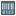• # Unclear text and examples

## Question related to missionCheckSum

I feel there's some inconsistency in this tasks description. see here: 1. From the rightmost input, traverse from right to left, and double the value for even/second character then in the example table we read:

```Character   :   0   1   2   3   4   5   6   7   8   9
Doubling    :   0   2   4   6   8   10  12  14  16  18
```

wich suggests every character has to be doubled, not the only the even ones.

Furthermore I would suggest that in the table containing the map point character lookup example you use the same numbers as the example and the final character table.

Some more reading brought some more unclearance about the mappoint generation: seeing this example

```    Reversed    :   T   M   9   3   1
Sum of digits   :   9   29  9   3   2   Total: 52
Final Character :   10 - ( 52 % 10 ) = 10 - 2 = 8
```

Here is detail for how we do it: T: ASCII of 84, 84 - 48 = 36, 36 * 2 = 72, and 7 + 2 = 9 M: ASCII of 77, and 77 - 48 = 29 9: from map point is, 9, or ASCII of 57, 57 - 48 = 9, 9 * 2 = 18, and 1 + 8 = 9 3: just 3, or ASCII of 51, and 51 - 48 = 3 1: from map point is, 2, or ASCII of 49, 49 - 48 = 1, and 1 * 2 = 2 Sum of digits is 52, since 9 + 29 + 9 + 3 + 1 = 52 Final character is 8, since 10 - ( 52 % 10 ) = 10 - 2 = 8 we see that the T,9 and 1 characters are doubled, but the description tells us from right to left every second/even one has to be doubled.15